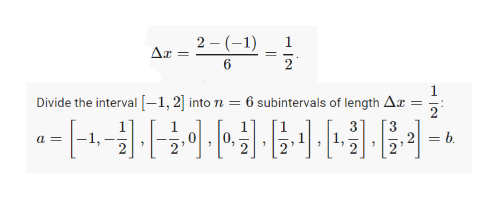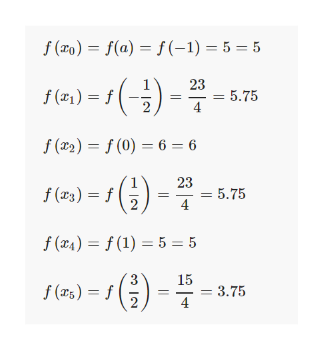# Find an approximation of the area of the region R under the graph of the function f on the interval [-1, 2]. Use n = 6 subintervals. Choose the representative points to be the left endpoints of the subintervals.f(x) = 6 - x2

Question
178 views

Find an approximation of the area of the region R under the graph of the function f on the interval [-1, 2]. Use n = 6 subintervals. Choose the representative points to be the left endpoints of the subintervals.
f(x) = 6 - x2

check_circle

Step 1

We have that a=−1, b=2, n=6help_outlineImage Transcriptionclose2 (1) 1 6 subintervals of length Ar 2 Divide the interval 1,2 into n -[+-130 036-11물이 b ,2 2 a= 2 fullscreen
Step 2

Then we evaluate the function ...help_outlineImage Transcriptionclosef(o)= f(a)= f(-1) = 5 = 5 23 = 5,75 4 f(e)3 f(x2)= f(0) 6 = 6 23 5.75 4 f() f (x4)= f(1) 5 = 5 15 3.75 4 f (ars)= f = 2 fullscreen

### Want to see the full answer?

See Solution

#### Want to see this answer and more?

Solutions are written by subject experts who are available 24/7. Questions are typically answered within 1 hour.*

See Solution
*Response times may vary by subject and question.
Tagged in